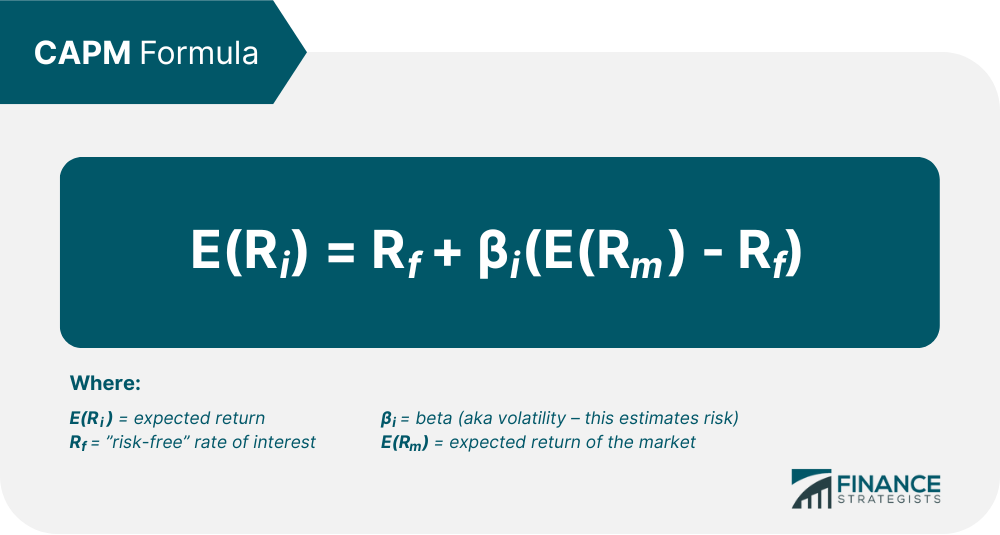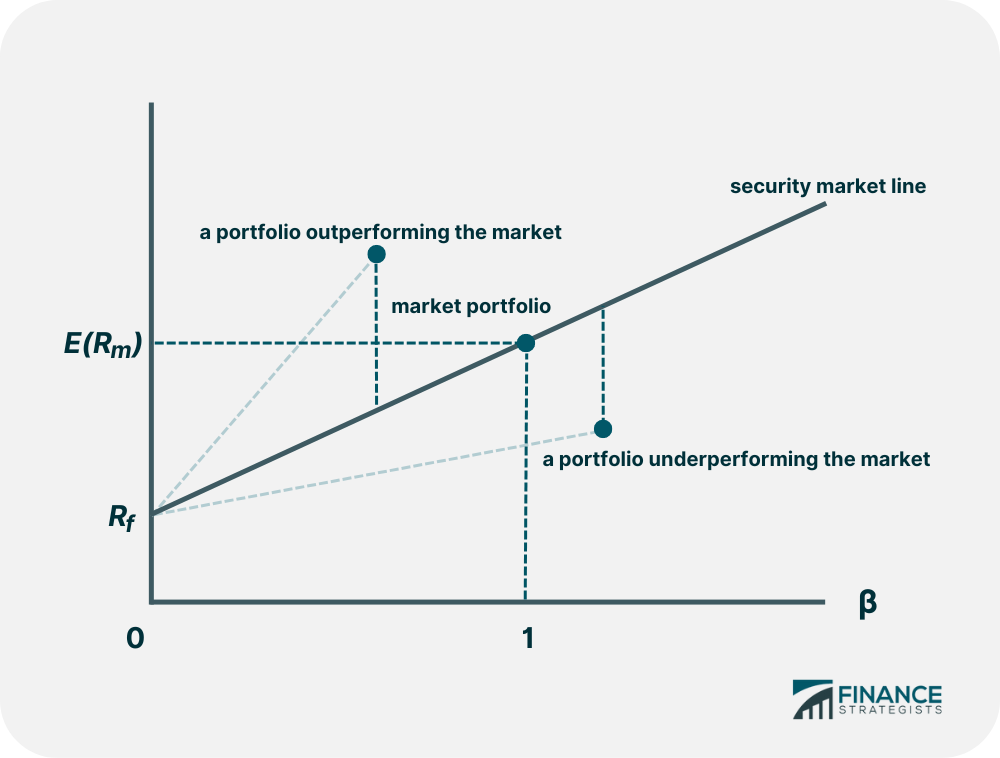# Capital Asset Pricing Model (CAPM)### Written byTrue Tamplin, BSc, CEPF® | Reviewed by Editorial Team

Updated on January 05, 2023

## Capital Asset Pricing Model (CAPM) Overview

The Capital Asset Pricing Model, or CAPM, calculates the value of a security based on the expected return relative to the risk investors incur by investing in that security. To calculate the value of a stock using CAPM, multiply the volatility, known as "beta," by the additional compensation for incurring risk, known as the "Market Risk Premium," then add the risk-free rate to that value.

## CAPM Formula

The formula for CAPM is as follows:In layman's terms, the CAPM formula is: Expected return of the investment = the risk-free rate + the beta (or risk) of the investment * the expected return on the market - the risk free rate (the difference between the two is the market risk premium).

For each additional increment of risk incurred, the expected return should proportionately increase.

If a security is found to have a higher return relative to the additional risk incurred, then the CAPM model suggests that it is a buying opportunity.

Like all valuation models, CAPM has its limitations since some assumptions it uses are idealistic. For example, Beta coefficients are unpredictable, change over time, only reflect systemic risk rather than total risk. Despite its shortcomings, this model is very popular for valuing securities.## CAPM FAQs

### What does CAPM stand for?

CAPM stand for “Capital Asset Pricing Model” and is a common valuation method for stocks.

### What is CAPM?

The Capital Asset Pricing Model, or CAPM, calculates the value of a security based on the expected return relative to the risk investors incur by investing in that security.

### How is CAPM calculated?

To calculate the value of a stock using CAPM, multiply the volatility, known as “beta,” by the additional compensation for incurring risk, known as the “Market Risk Premium,” then add the risk-free rate to that value.

### What is the formula for CAPM?

Expected return of the investment = the risk-free rate + the beta of the investment times the expected return on the market – the risk free rate which is the market risk premium.